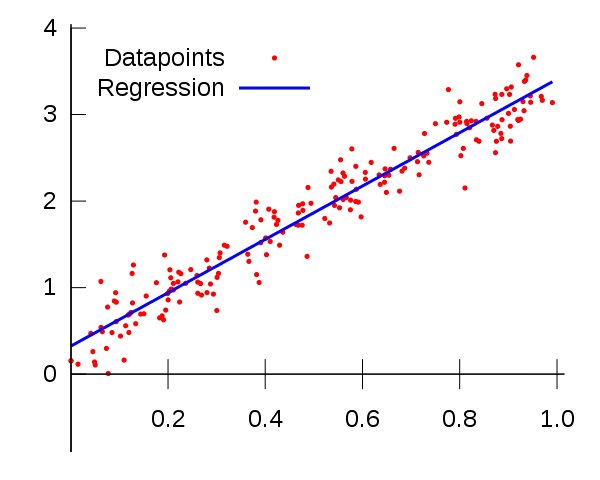## Relation between statistics and machine learning

Excerpt from

https://www.jiqizhixin.com/articles/2019-04-24-16

May share similar methods, but main difference is their purpose. Machine learning models aim at more accurate prediction. Statistic models aim at infering relations between variables.

Machine learning sacrifices interpretability, statistics model may predict but not so satisfactory.

A exampleLinear regression is a statistics method. Through the same linear regression method, we can train a linear classifier, we can also build a regression model by minimum least squares. The former needs the step of training, which means it requires a training set, then it will be tested on a test set to evaluate its performance. In machine learning, the ultimate goal is reaching a high accuracy. For the latter, we will assume it is a linear regressor with gaussian noise, and try to find a line to minimize the mean square error of all the data. The purpose of modeling is to describe the relation between variables and output, instead of making prediction. We can use this model for prediction, but model evaluation is not test set performance, but the robustness and signifance of parameters in the model.

An interesting table

 Glossary（） Machine   learning Statistics network, graphs model weights parameters learning fitting generalization test set performance supervised learning regression/classiﬁcation unsupervised learning density estimation, clustering large grant = \$1,000,000 large grant = \$50,000 nice place to have a meeting:     Snowbird, Utah, French Alps nice place to have a meeting:     Las Vegas in August